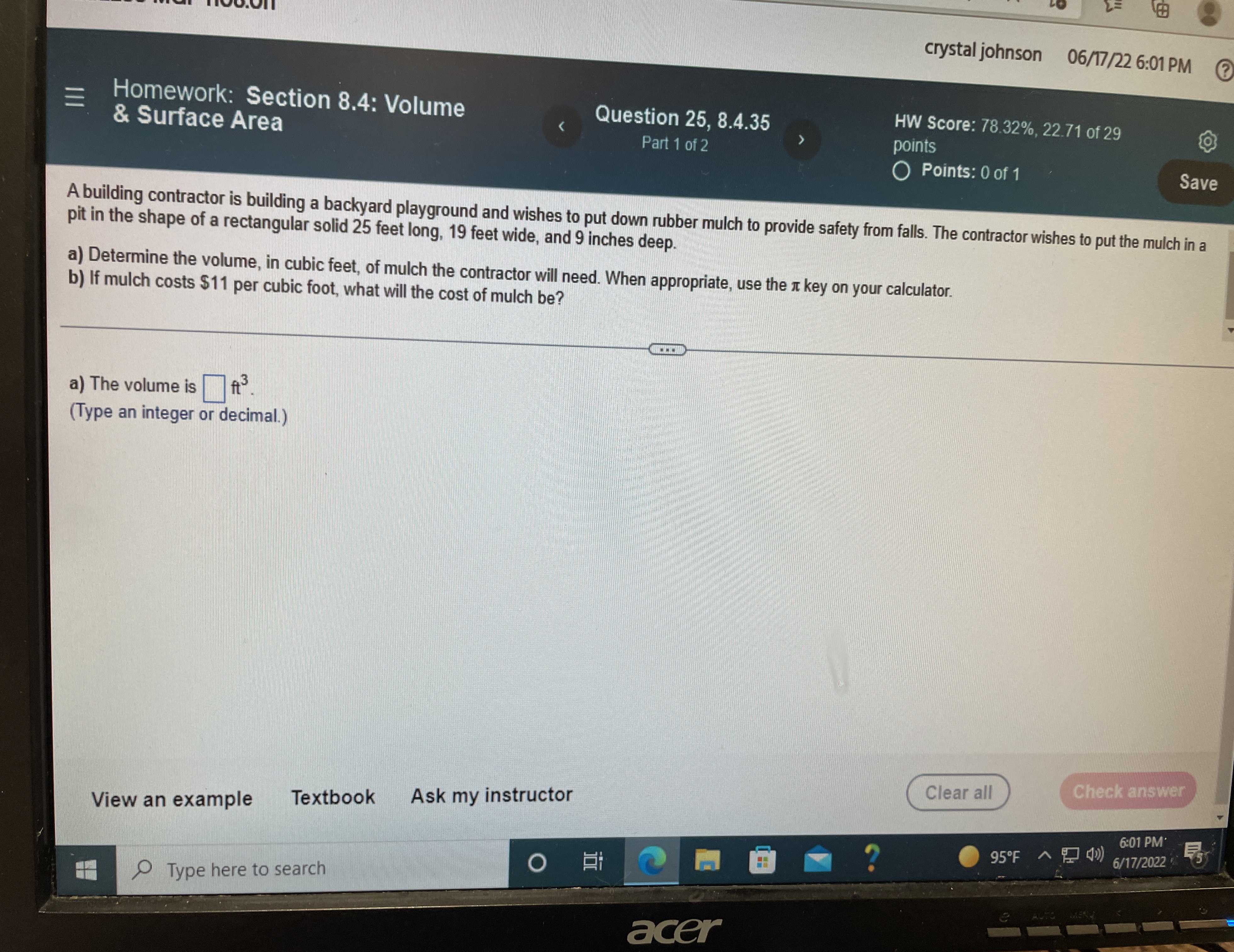### Still have math questions?

Trigonometry
QuestionA building contractor is building a backyard playground and wishes to put down rubber mulch to provide safety from falls. The contractor wishes to put the mulch in $$0$$ of $$1$$ pit in the shape of a rectangular solid $$25$$ feet long, $$19$$ feet wide, and $$9$$ inches deep.

a) Determine the volume, in cubic feet, of mulch the contractor will need. When appropriate, use the $$\pi$$ key on your calculator.

b) If mulch costs $$\ 11$$ per cubic foot, what will the cost of mulch be?

a) The volume is $$\square ft ^ { 3 }$$ .

(Type an integer or decimal.)